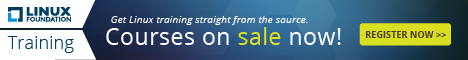<< View All Computer Tips

Added On: 22/10/18   |    Category: Vanilla Javascript    |    Number of Views: 1076

## Generate a random number between 1 and 5 using Math.floor and Math.random commands in Javascript    Tweet

The code below generates a random number between 1 and 5

Create a new .html document on your desktop, and rename the file example3.html
Open the document in your text editor and copy and paste the following code.

<script>
var random_number = Math.floor(Math.random() * 5 + 1);
document.write(random_number);
</script>

Example 1: Using document write() in javascript
Example 2: Using document.getElementByID() in javascript to send form data
Example 3: Generate a random number between 1 and 5 using Math.floor and Math.random commands in Javascript